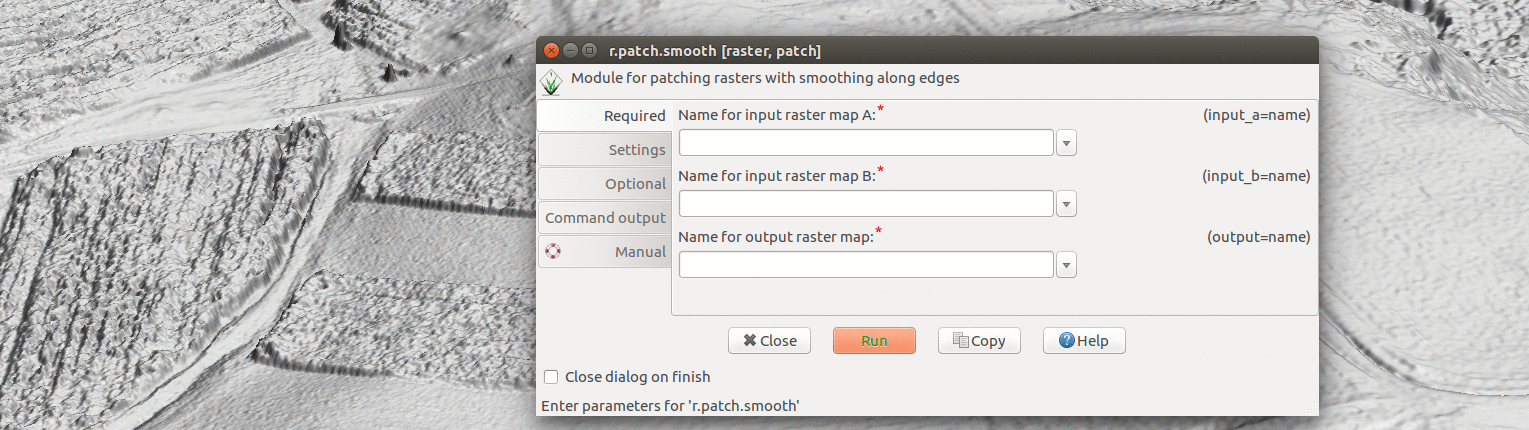# Seamless fusion of high-resolution DEMs from multiple sources

## Background

In partnership with NextGen Air Transportation System (NGAT)
Center for Geospatial Analytics (CGA) collects, processes and analyses UAS data for research and education purposes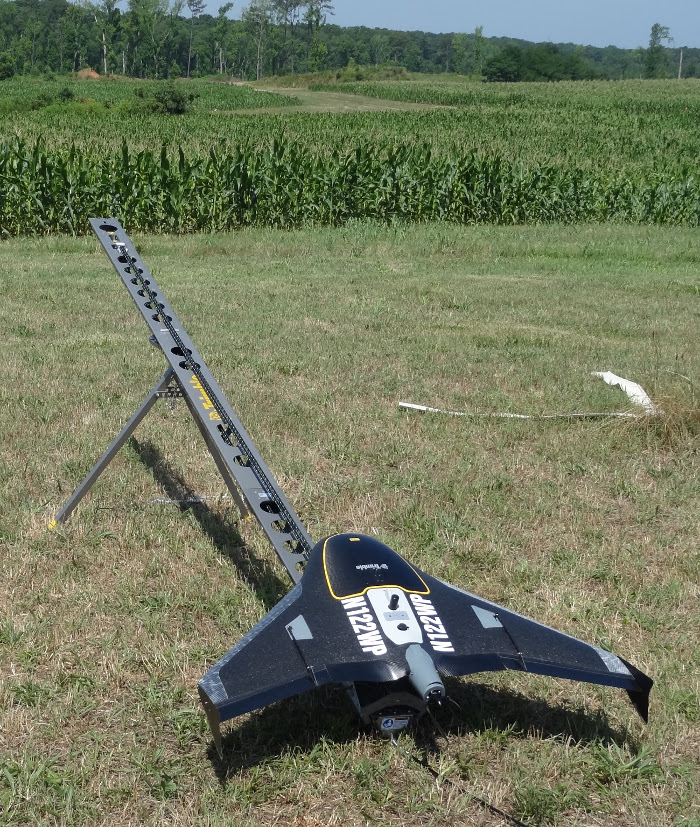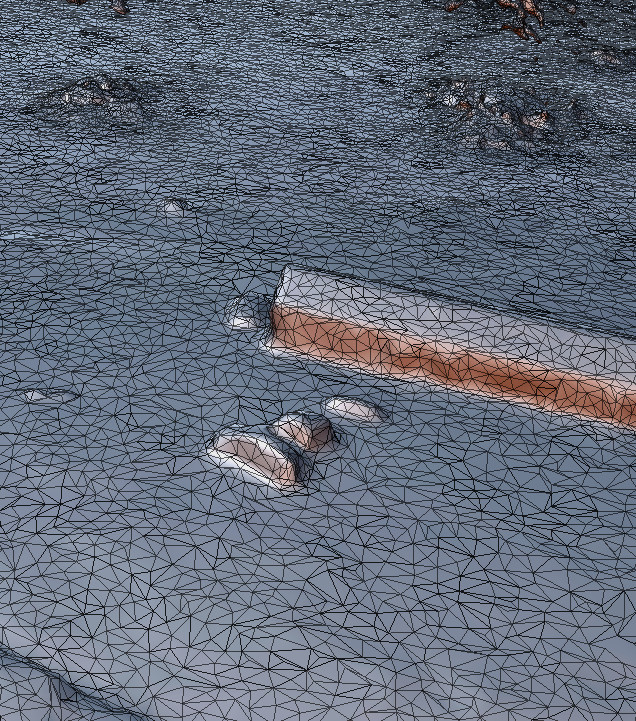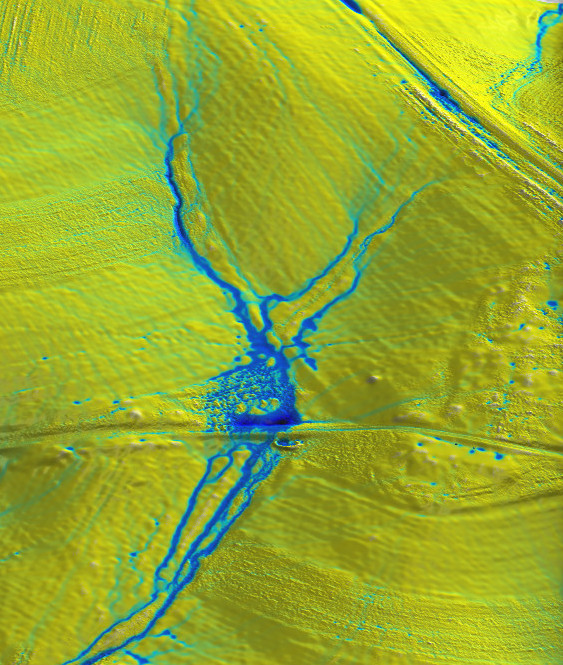## UAS & lidar course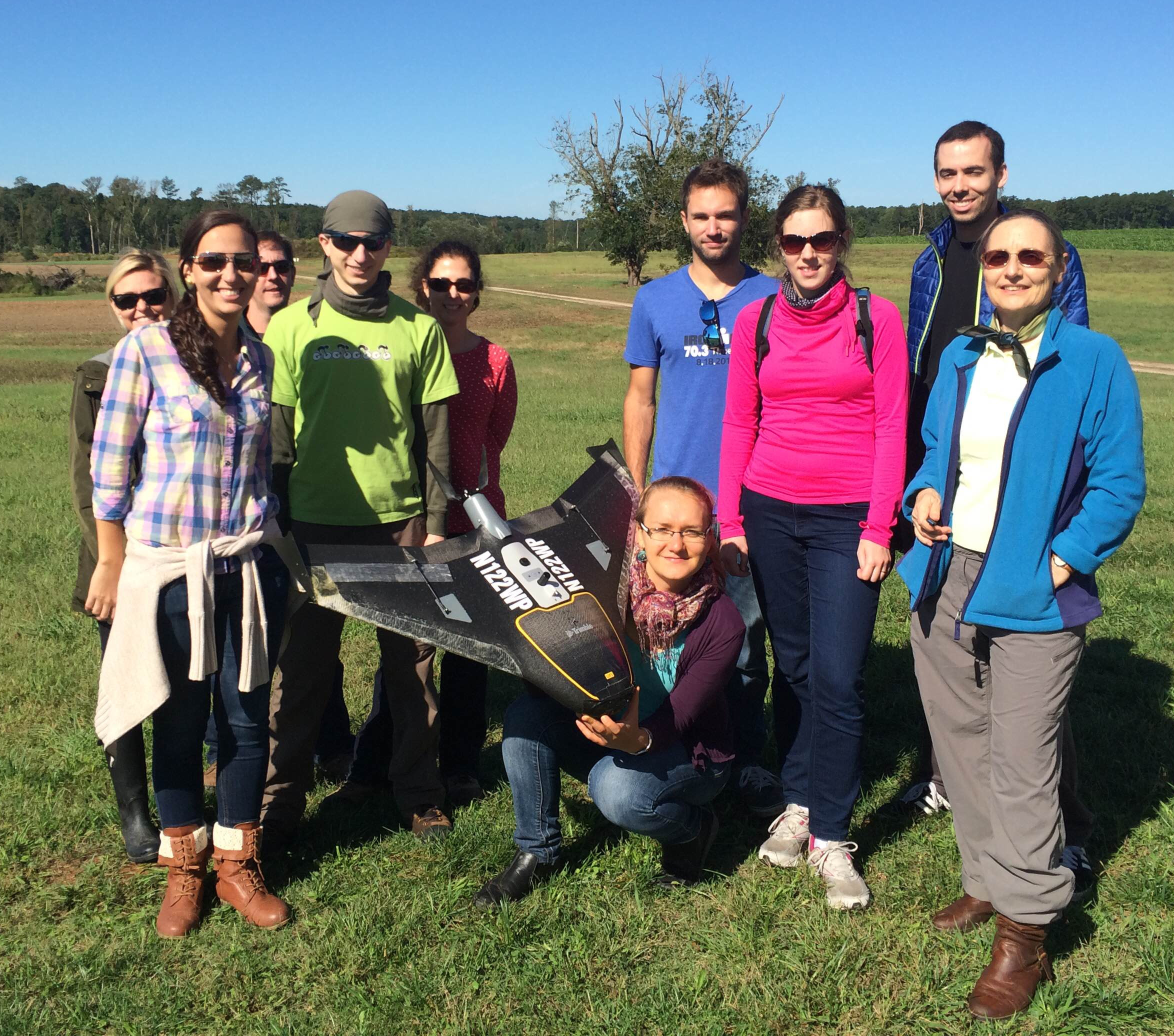Data collection and analysis:

• flight planning, characteristics of UAS and lidar data, errors, point cloud processing and DEM reconstruction, landscape processes

Student projects:

• mapping soil erosion, beaver dam impact
• crop growth monitoring, forest structure

Software:

• GIS, SfM software (AgiSoft, OpenDroneMap), Python scripting

## Lake Wheeler Road Field Laboratorystudy area

#### 2015 - 2017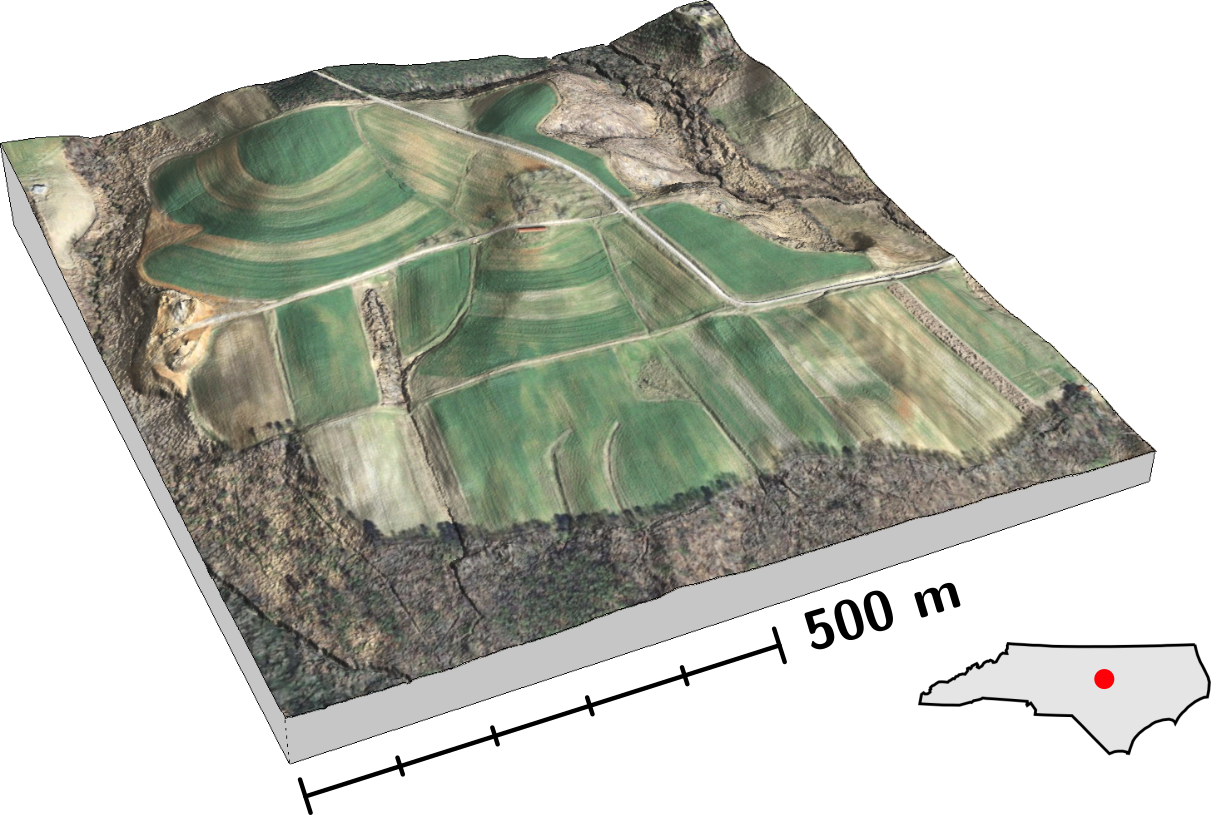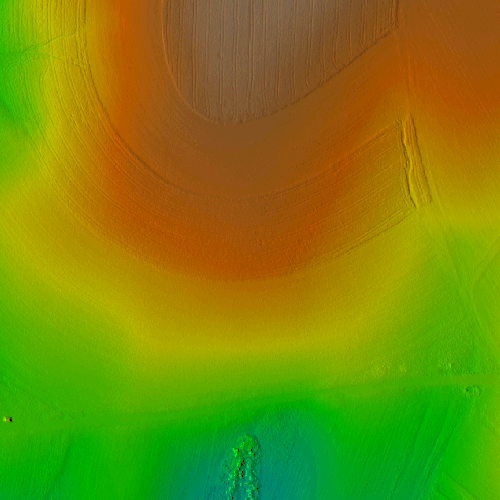## Data analysis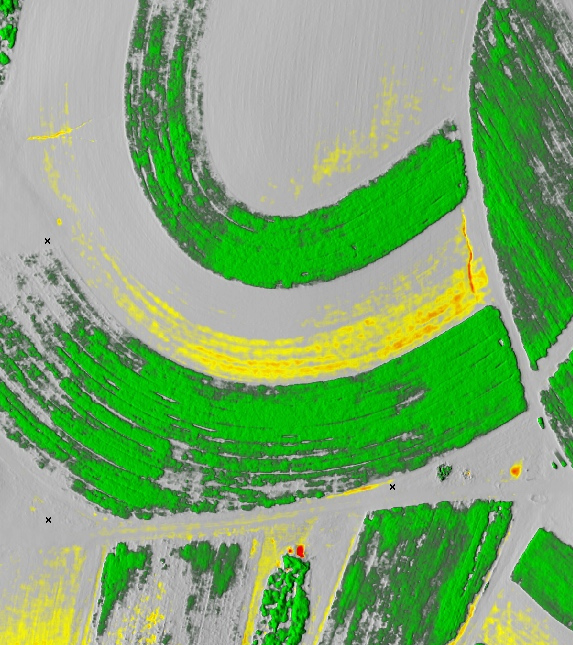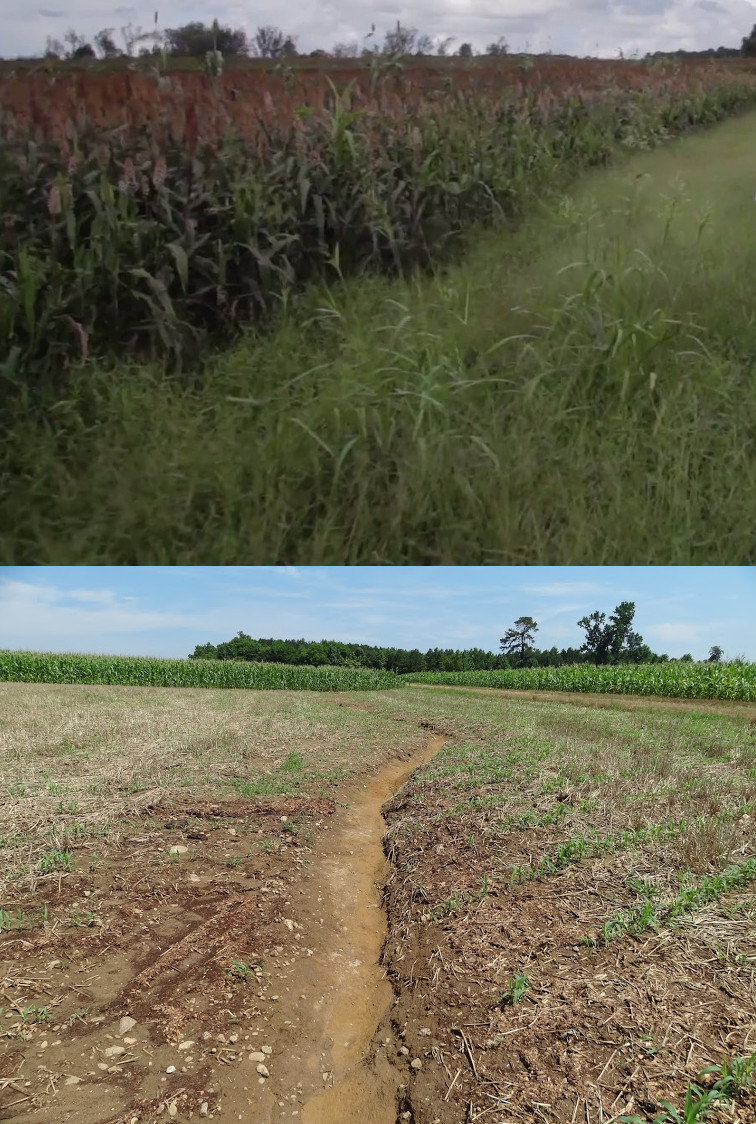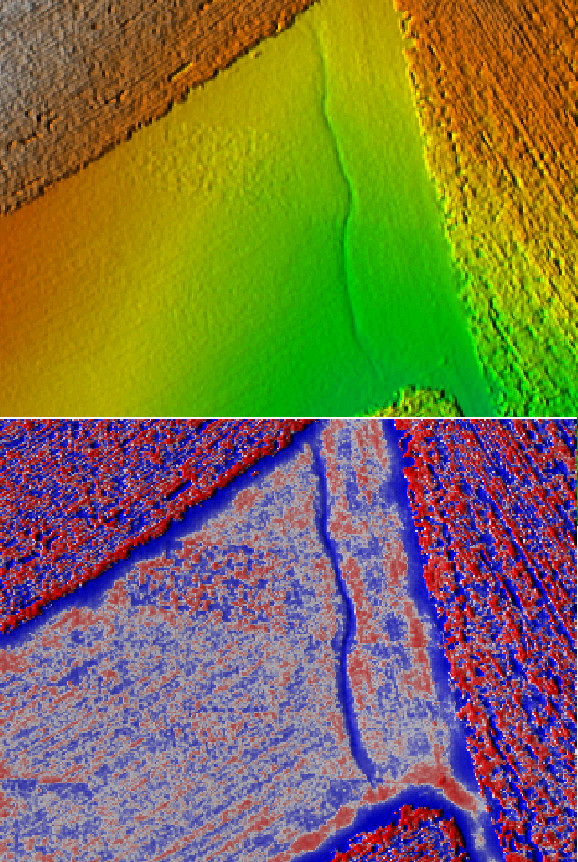change detection processes microtopography

## Modeling landscape processes

### at a microtopographic level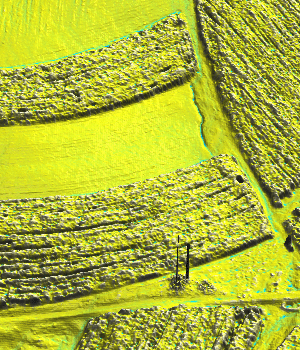water flow erosion landscape evolution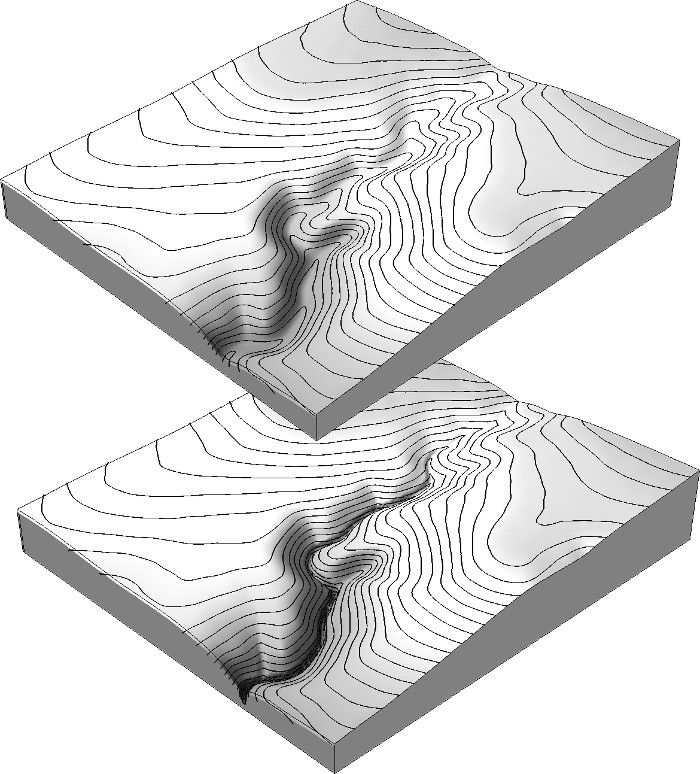### UAS + SfM

• very dense point clouds (hundreds per square meter)
• simple to repeat data collection
• smaller area
• some areas are excluded
• difficult to extract ground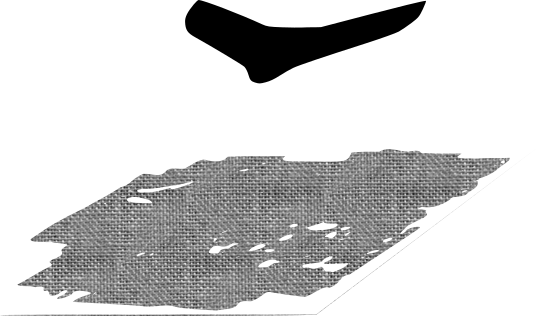### UAS + SfM

• very dense point clouds (hundreds per square meter)
• simple to repeat data collection
• smaller area
• some areas are excluded
• difficult to extract ground### Airborne lidar

• moderately dense point clouds (several points per square meter)
• expensive, can't be repeated often
• large area
• provides ground data## DEM fusion

#### aka DEM mosaicking, merging, patching

Motivation:

• boundaries of UAS-based DEM and modeled phenomenon don't match (e.g. watersheds)
• need to replace vegetated areas with ground
• updating part of older DEM with newer DEM

What we need:

• simple and robust raster- and GIS-based method
• continuous DEM with smooth transition between different DEM sources

## Problem

 new = if(isnull(UAS), lidar, UAS)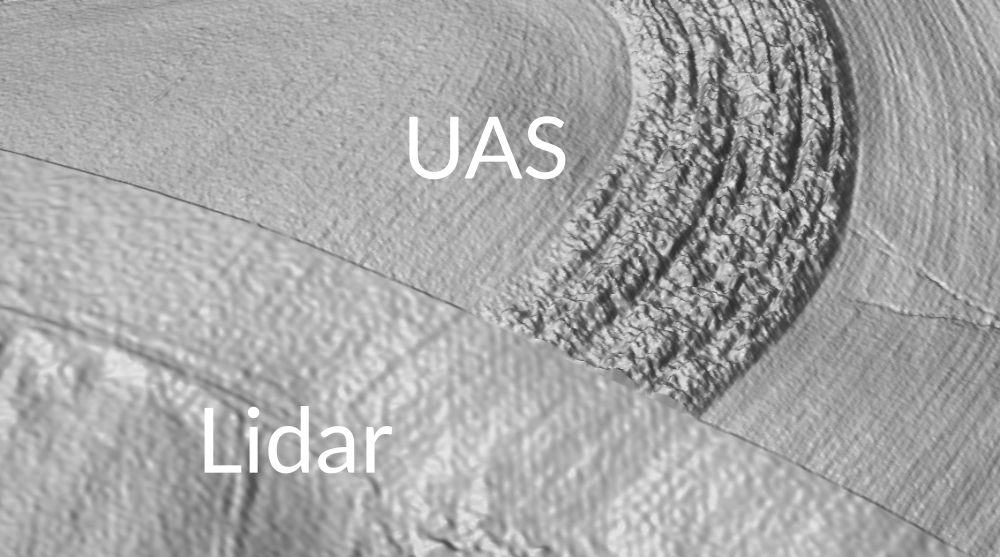## Water flow on patched DEM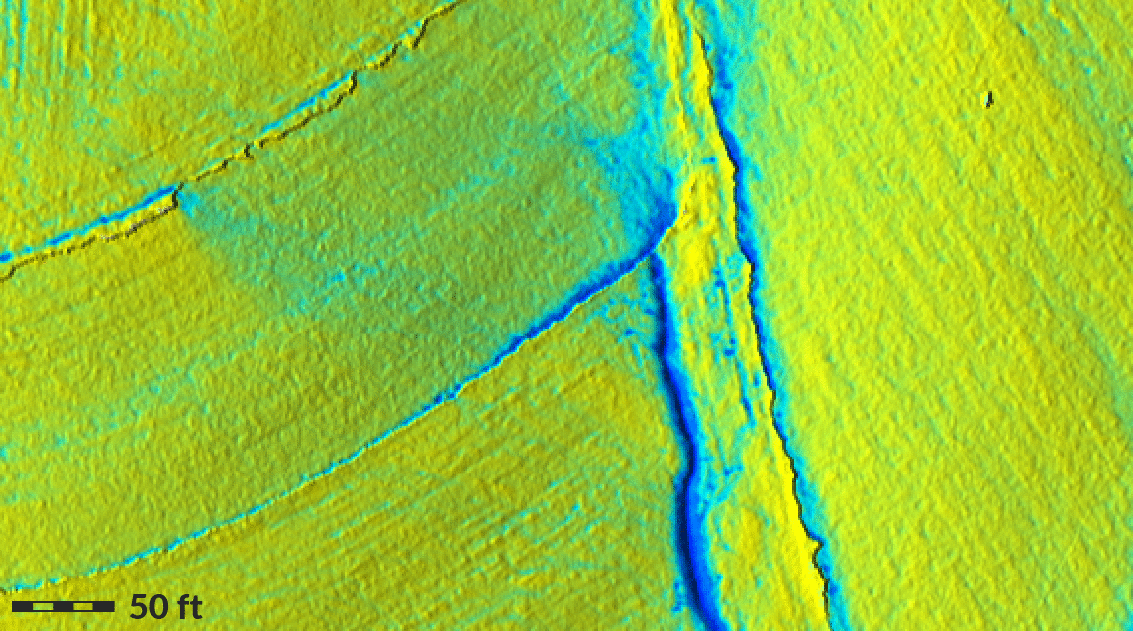## Water flow on fused DEM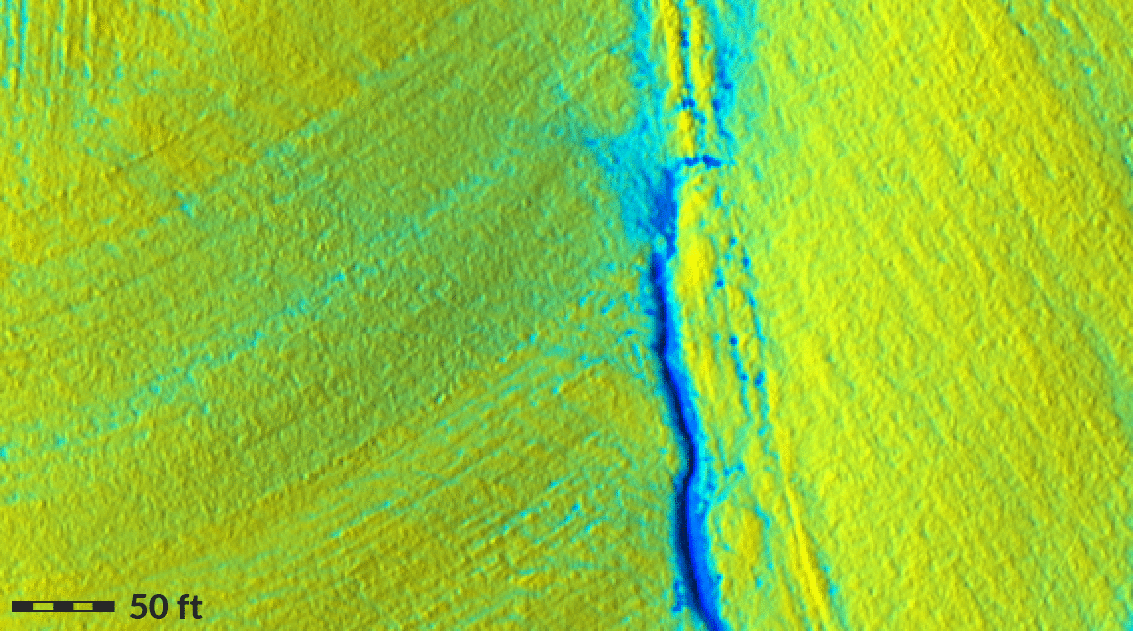## Fusion method (v1)

Compute weighted average of DEM $A$ and $B$ on their overlap of width $s$ with weights given by the distance to the edge of DEM $A$.

What do we need?

• two DEMs of the same resolution and aligned
• raster algebra
• euclidean distance tool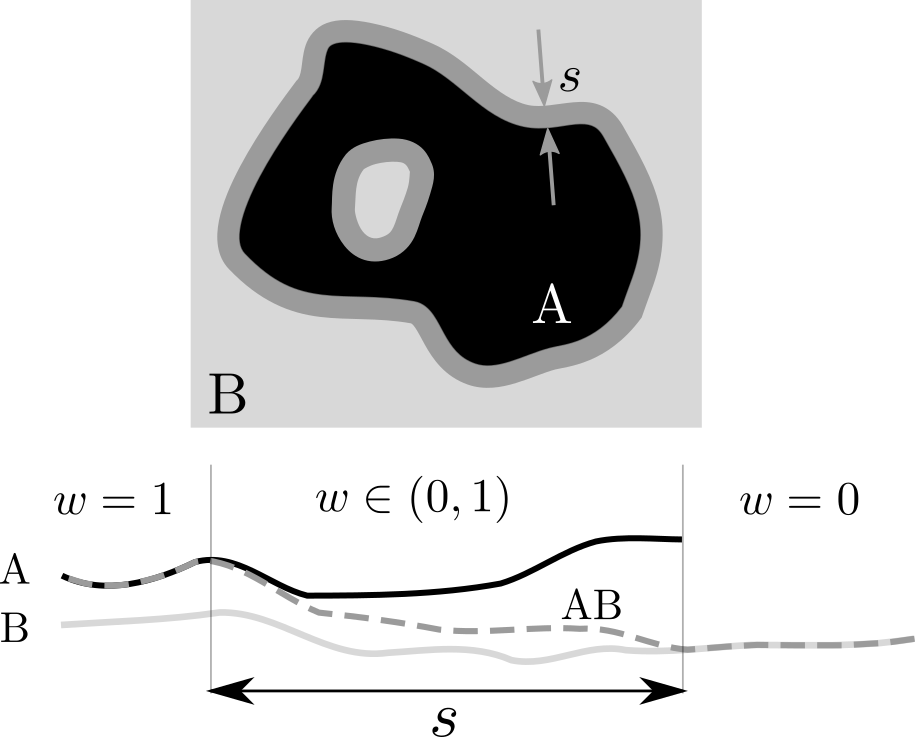## Step 1.

 Distance from the edge of DEM $A$ inwards.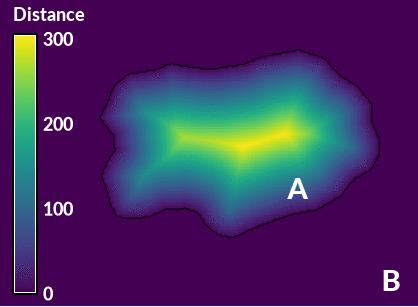GRASS GIS:

r.grow.distance -n input=A distance=distance


## Step 2.

Linear combination of elevation surfaces $z_{A}$ and $z_{B}$ with weights given by distance $d$ and overlap width $s$ : $$z_{AB} = z_{A} w + z_{B}(1 - w), \quad\quad w = f(s, d) = \begin{cases} \frac{d}{s} & 0 \leq d < s \\ 1 & d > s \end{cases}$$

Raster algebra expression for $s = 10$ m:

if (distance > 10, A,
if (distance == 0, B,
(1 - distance / 10) * B + (distance / 10 * A)
)
)


## Smoothing parameter $s$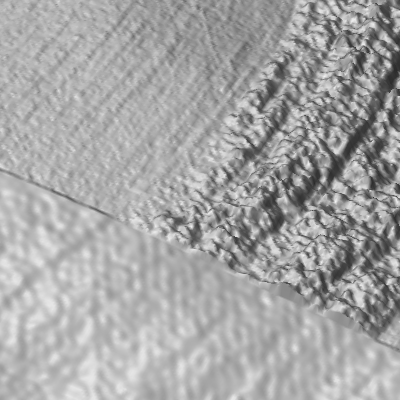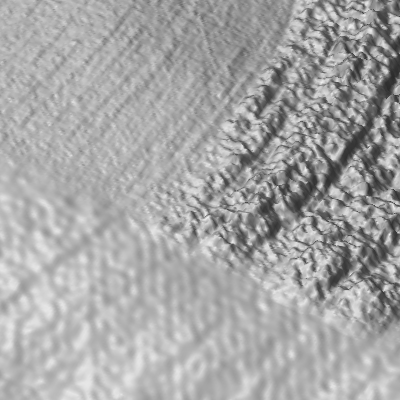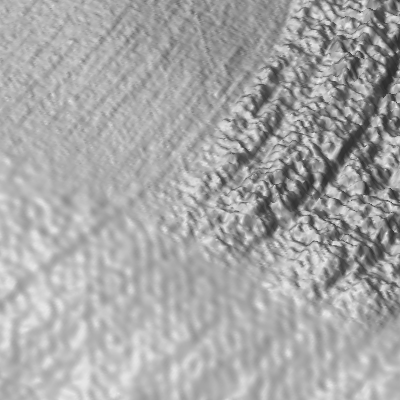0 m 5 m 10 m 20 m

## Fusion method (v2)

By taking into account spatially variable differences $\Delta z$ between DEMs $A$ and $B$ along the overlap:

• we get more gradual transition where differences are high
• we preserve subtle features of both DEMs where differences are small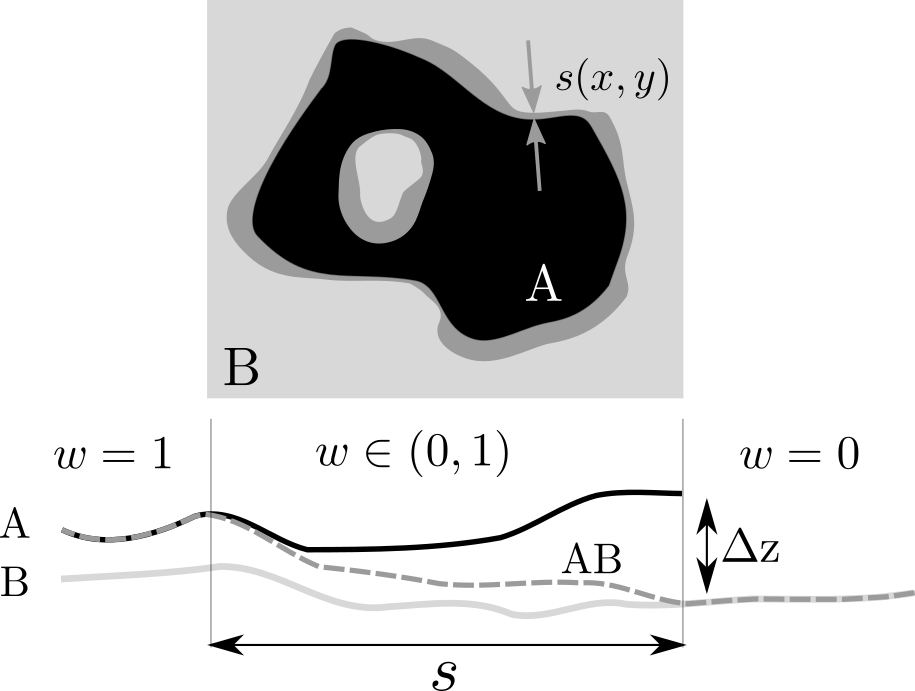### Fusion method (v2): example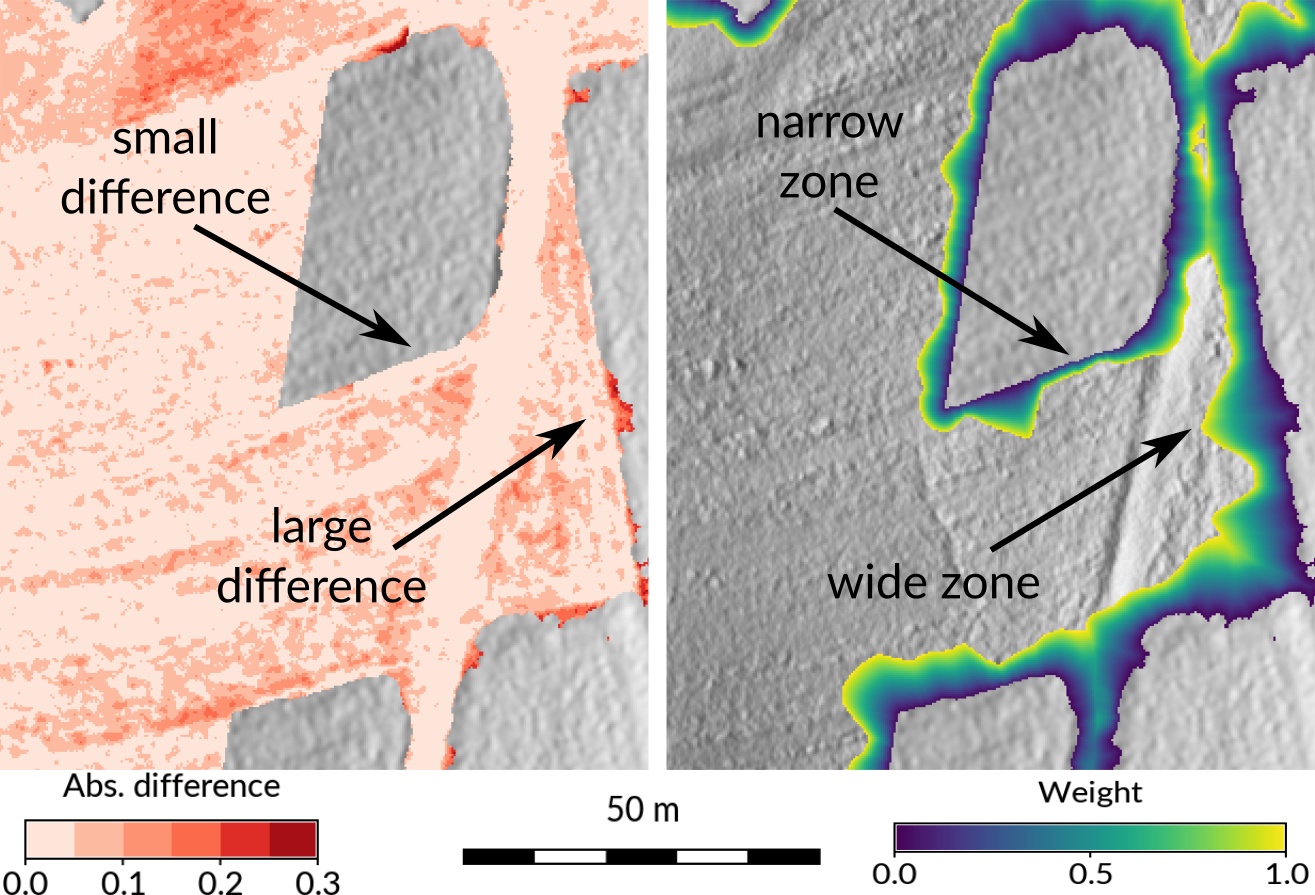## Fusion method (v2): steps

1. Compute distance from DEM $A$ inwards (distance tool).
2. Derive absolute difference between A and B, and from that extract one-pixel-wide edge as raster (raster algebra).
3. Derive raster where each cell has the value of the nearest cell from that edge raster (distance tool).
4. Combine the distance raster from step 1) and the raster with differences from step 3) into raster representing weights (raster algebra).
5. Compute linear combination of DEMs using this raster of weights (raster algebra).

## Use case: Tangible Landscape

• tangible geospatial interface
• couples a malleable physical model with a digital landscape through a cycle of real-time scanning, analysis, and projection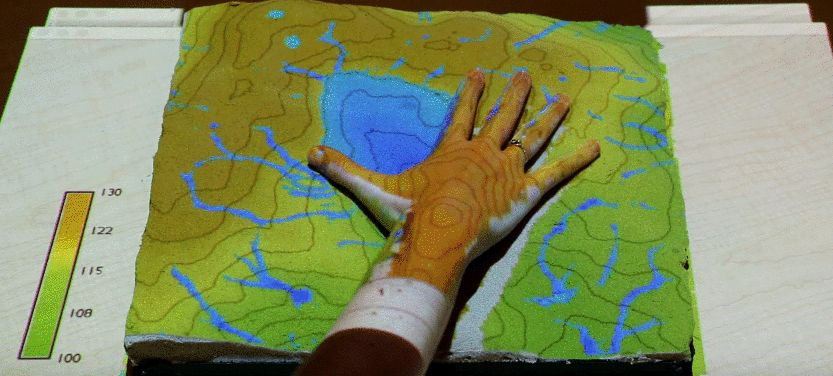### Fusion of lidar- and kinect-based DEMs

• simulating and testing methods for controlling storm water flow
• we need smooth fusion to let simulated water flow into and out of the model
• real-time fusion of a georeferenced, physical sand model scanned continuously by Kinect with a lidar-based DEM of the surrounding watershed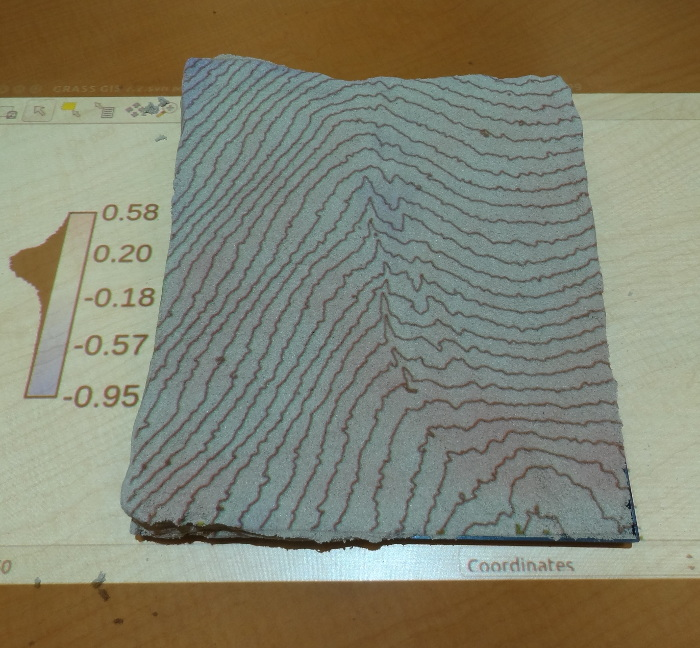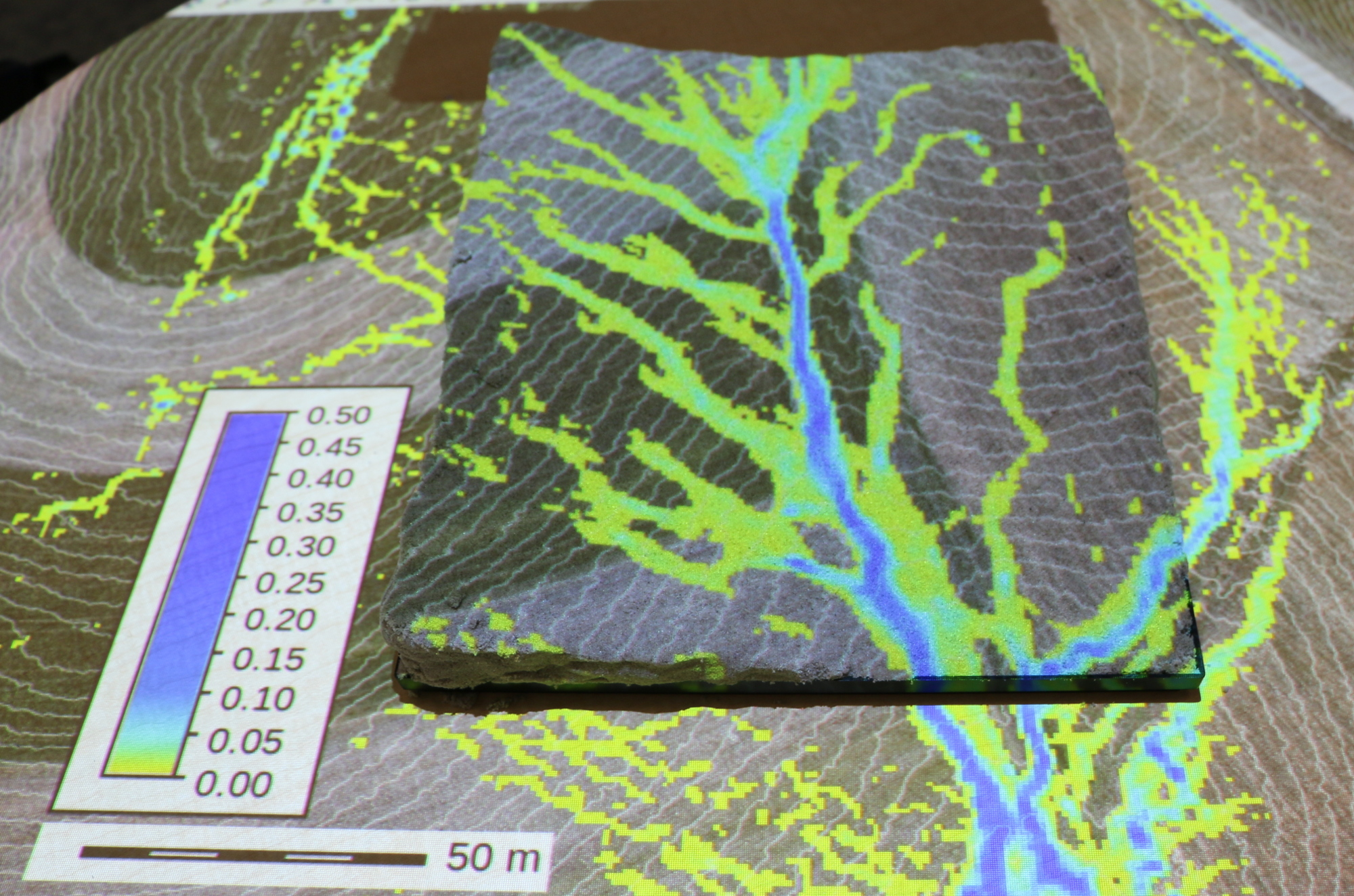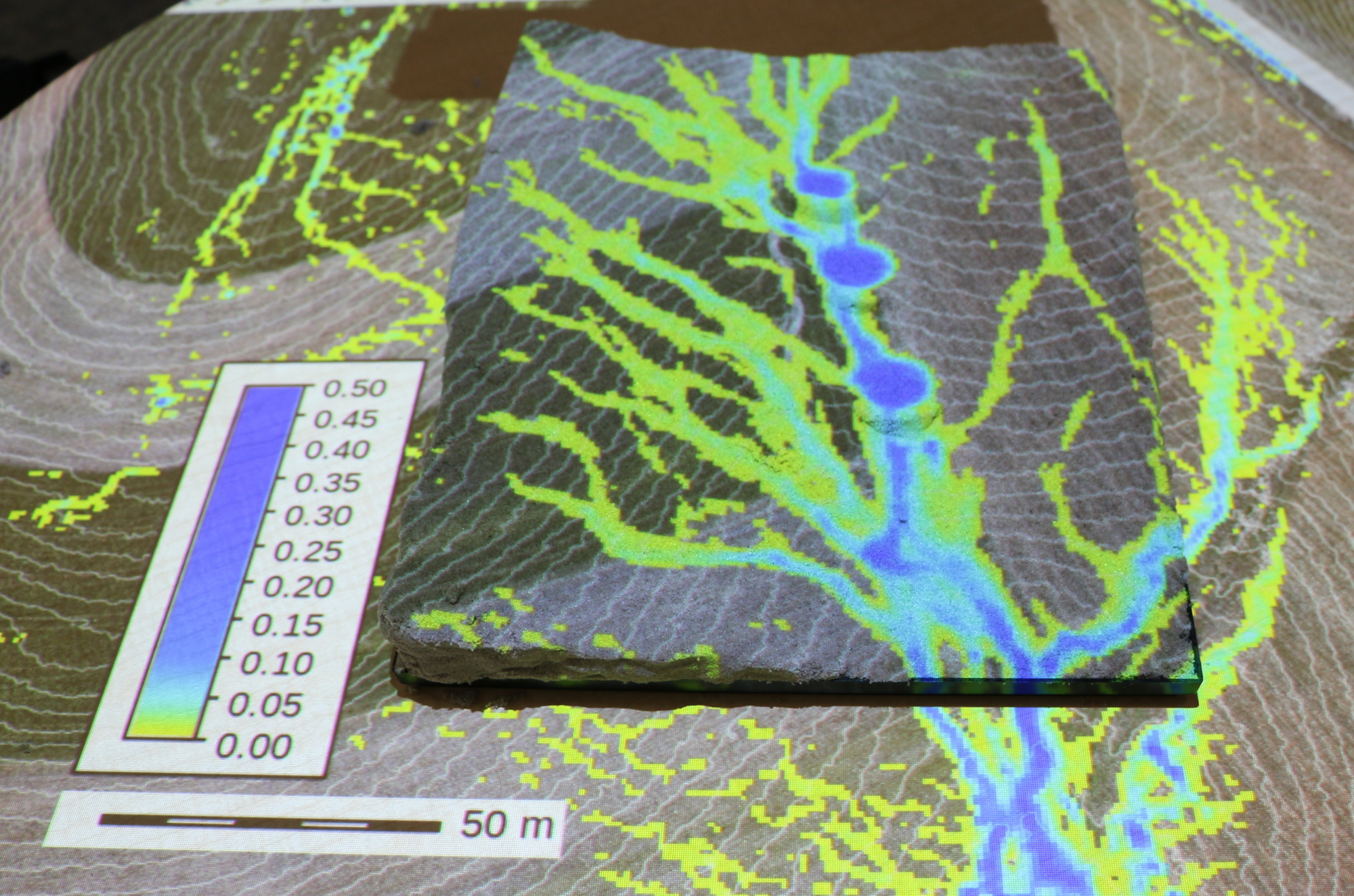sand model before change modified landscape

## Resources

• Method will be published in Open Geospatial Data, Software and Standards as open access article
• GRASS GIS addon r.patch.smooth g.extension r.patch.smooth url=https://github.com/petrasovaa/r.patch.smooth
• Sample dataset for testing: geospatial.ncsu.edu/osgeorel/data.html